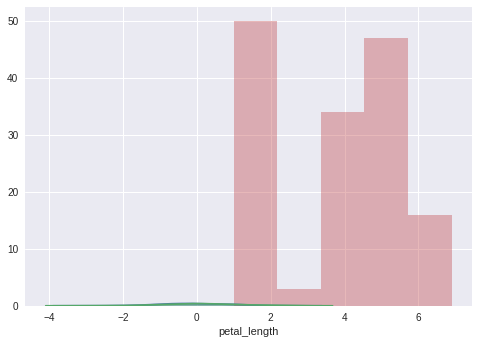# Seaborn - Histogram¶

## Histograms¶

A histogram represents the data distribution by forming bins along the range of the data and then drawing bars to show the number of observations that fall in each bin.

Seaborn comes with some datasets and we have used few datasets in our previous chapters. We have learned how to load the dataset and how to look up the list of available datasets.

Seaborn comes with some datasets and we have used a few datasets in our previous chapters. We have learned how to load the dataset and how to look up the list of available datasets.

## Visualizing the distribution of a dataset¶

When dealing with a set of data, often the first thing you’ll want to do is get a sense of how the variables are distributed. This chapter of the tutorial will give a brief introduction to some of the tools in seaborn for examining univariate and bivariate distributions. You may also want to look at the categorical plots chapter for examples of functions that make it easy to compare the distribution of a variable across levels of other variables.

In :
import pandas as pd
import seaborn as sb
from matplotlib import pyplot as pltSunny Solanki